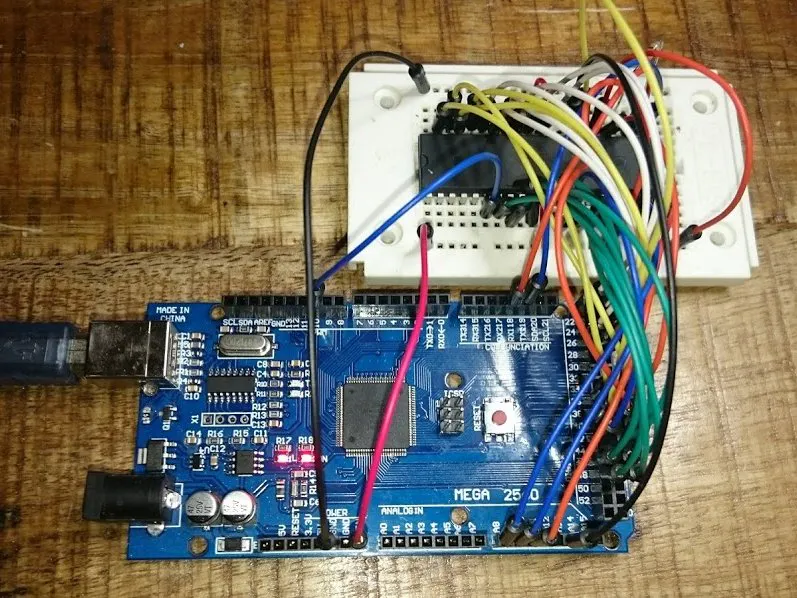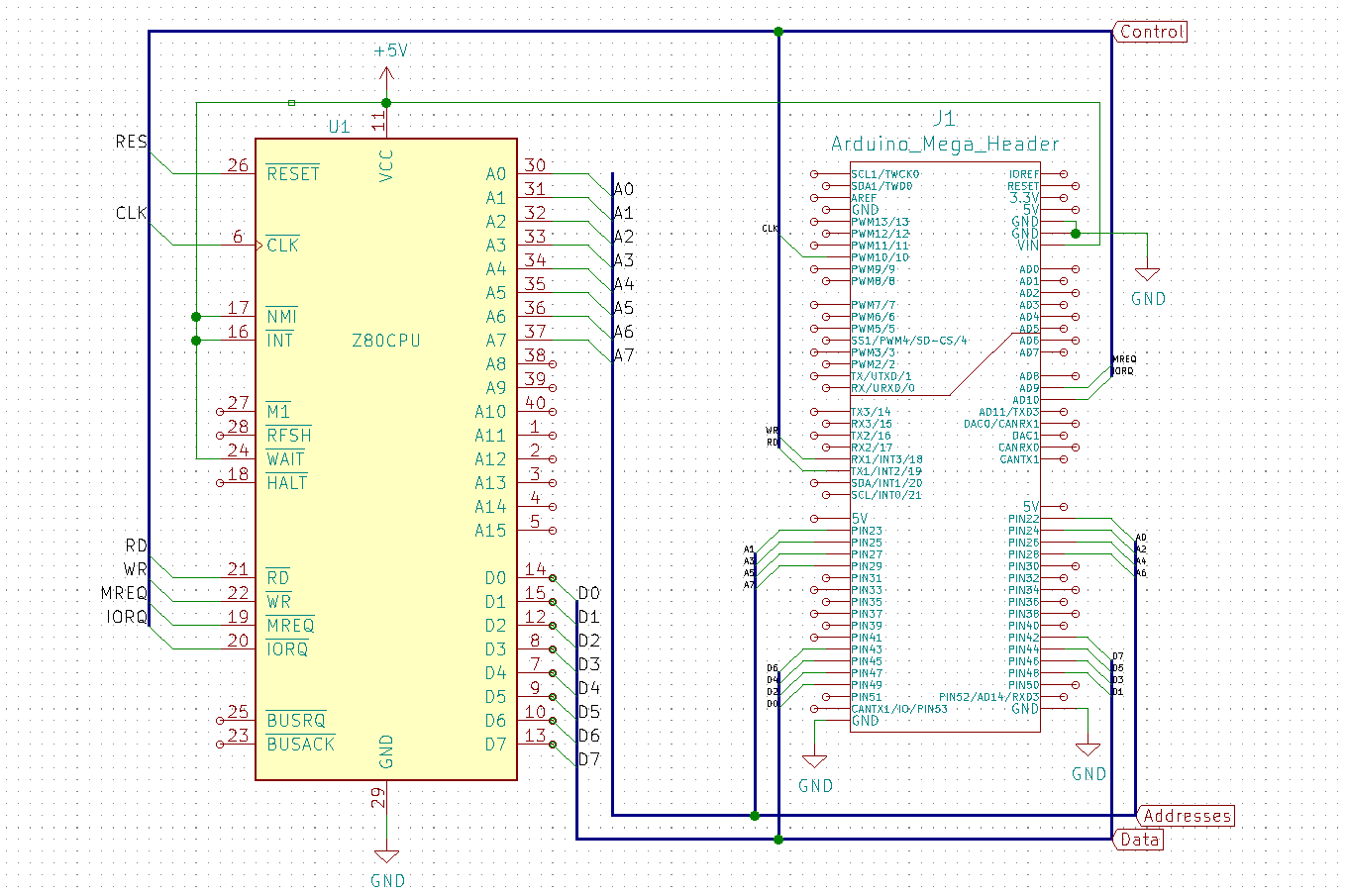# Retro Computing with Arduino Mega and a Z80 processor © CC BY-SA

Brought to the marked in 1976, Zilog's Z80 was the most widespread processor in the '80s until the 2000s. Let's bring it to life again!

• 14,971 views
• 39 respects

## Apps and online services

One day when I tidied up my cellar, I found a Z80 chip from an old computer. In the '80s and '90s, this processor was very popular, and I dare to say the most popular processor ever. So I got the idea to breathe new life into it.

The Z80 has a tinker-friendly 40-pin DIP housing, but unlike modern tinker-friendly Processors like the ATmegas, there is no built-in RAM, program flash or any peripherals. It has only the raw core functionality of every Processor, which is to make basic calculations on some bits and bytes.

With a bunch of jumper wires, a small breadboard and an Arduino Mega that emulates ROM, RAM and serial IO, I made a fully fledged Z80-based computer!

This is just an academical fun project without any practical relevance, but it teaches you how microprocessors still work in detail!

Watch the video, if you are interested in the details!

## Code

##### z80-test.inoC/C++
Sketch for interaction between Arduino and Z80 CPU
```#include "memory.h"

/*Wiring:
Arduino Pin 22..29   <--->   Z80 A0..A7

Data Lines:
Arduino Pin 49..42   <--->   Z80 D0..D7

Control lines:
Arduino Pin 10  <--->  Z80 Pin 6 (CLK)
Arduino Pin 19  <--->  Z80 Pin 21 (RD)
Arduino Pin 18  <--->  Z80 Pin 22 (WR)
Arduino Pin A9  <--->  Z80 Pin 19 (MREQ)
Arduino Pin A10 <--->  Z80 Pin 20 (IORQ)
Arduino Pin 40  <--->  Z80 PIN 26 (RESET)
*/

//Working memory
uint8_t memory;

//Z80 Out
//Port D
#define RD_PIN PD2 //(INT2 - Arduino Pin 19) Z80 Pin21
#define WR_PIN PD3 //(INT3 - Arduino Pin 18) Z80 Pin 22
#define RD    ((PIND & (1 << RD_PIN)) == 0)
#define WR    ((PIND & (1 << WR_PIN)) == 0)
#define PORT_MASK_D ~((1 << RD_PIN) + ( 1 << WR_PIN));

//Port K
#define MREQ_PIN PK1 //(PCINT17 - Arduino Pin A9) Z80 Pin 19
#define IORQ_PIN PK2 //(PCINT18 - Arduino Pin A10) Z80 Pin 20

#define MREQ  ((PINK & (1 << MREQ_PIN)) == 0)
#define IORQ  ((PINK & (1 << IORQ_PIN)) == 0)

#define ADDR_HI (PINC & (PC0 | PC1)) //A8..A9

//Z80 In
//PortB
#define CLK_PIN PB4 //(OC2A) Z80 Pin 6

//Port G
#define INT_PIN PG0 //Z80 Pin 16, Arduino Pin 41
#define RST_PIN PG1 //Z80 PIN 26, Arduino Pin 40
#define WAIT_PIN PG2 //Z80 Pin 24, Arduino Pin 39
#define RESET(b)  b == 0 ? PORTG |= (1 << RST_PIN) : PORTG &= ~(1 << RST_PIN);
#define PORT_MASK_G (1 << INT_PIN) + (1 << RST_PIN) + (1 << WAIT_PIN);

// the setup function runs once when you press reset or power the board
void setup() {
Serial.begin(115200);
const long fClk = 1E5;
//Serial.begin(256000);
DDRA = 0x00; //Port A (Addr Low) input
DDRC = 0x00; //Port C (Addr Hi) input
DDRK = 0x00; //Port K Input (Z80 sysctl out)
DDRL = 0xff; //Data Bus
PORTL = 0x00;

//Pin Interrupts
EICRA |= (1 << ISC31) + (0 << ISC30) + (1 << ISC21) + (0 << ISC20);
EIMSK |= (1 << RD_PIN) + (1 << WR_PIN);

//Clock Timer2
DDRB |= (1 << CLK_PIN); //Output
TCCR2A = (0 << COM2A1) + (1 << COM2A0); //Toggle OC2A on Compare Match
TCCR2A |= (1 << WGM21); //CTC Mode
TCCR2B = (0 << CS22) + (0 << CS21) + (1 << CS20); //No Prescaling
OCR2A = 16E6 / (2 * fClk) - 1;

memset(memory, 0, sizeof(memory));
memcpy(memory, mem, sizeof(mem));

RESET(1);
delay(1);
RESET(0);
}

// the loop is empty, because this is interrupt-driven
void loop() {}

inline uint8_t DATA_GET() {
DDRL = 0;
return PINL;
}

//Write data to Data Bus
inline void DATA_PUT(uint8_t d) {
DDRL = 0xff;
PORTL = d;
}

if (MREQ) {
}
}

ISR(INT3_vect) {//CPU Writes
if (MREQ) {
}
else {//IORQ
Serial.print((char)DATA_GET());
}
}
```
##### memory.hC/C++
Header file with "Hello World" machine program
```#ifndef MEMORY_H
#define MEMORY_H

uint8_t mem[] = {
0x21, 0x0a, 0x00, //ld hl, ??
0x0e, 0x00, //ld c, 0
0x06, 0x0c, //ld b, 12
0xed, 0xb3, //otir
0x76, //halt
'H','e','l','l','o', ' ','W','o','r','l','d','\n'
};

#endif
```

## Schematics#### Similar projects you might like

Project in progress by Pigeon-Kicker

• 3,380 views
• 12 respects

Project tutorial by NZDoug

• 11,416 views
• 70 respects

#### TeleBall Retro Handheld Game Console

Project tutorial by sy2002

• 9,001 views
• 24 respects

#### Marco Polo || Mega Route Logger

Project showcase by Boaz Lawnce

• 1,652 views
• 5 respects

#### Mega Solar Tracker

Project in progress by Team Trouble

• 16,864 views